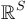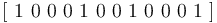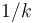Continuous encoding of categorical variables trick

Definition

The continuous encoding of categorical variables trick proceeds as follows. Consider a categorical variable$v$ that can take any value from a fixed finite set$S$.

Feature set interpretation

For each value in$S$, define a binary feature whose value is 1 if$v$ equals that value and 0 otherwise. We get a total of$|S|$ features (i.e., as many features as the size of$S$) corresponding to the different elements of$S$. This collection of features is called a feature family.

Moreover, because the categorical variable$v$ can take only one value within$S$, it is true that for any example, the values of the features from that family on that example include one 1 and the rest 0s.

Vector interpretation

We can think of the continuous encoding as an embedding of the (discrete) finite set$S$ into the finite-dimensional vector space$\R^S$, where each element of$S$ gets sent to the corresponding standard basis vector for that coordinate.

What this means for encoding examples

Direct encoding of examples

Consider a machine learning problem where each training example has a bunch of features that are categorical variables. For example, to each single item purchase from an e-commerce website, we may associate categorical variables such as:

1. The user ID of the buyer. Let's say there are three users, with IDs Alice, Bob, and Carol.
2. A unique identifier for the inventory item purchased. Let's say there are two items that can be bought: headphones and USB flash drives, and that a single purchase can only involve buying one inventory item.
3. The location that the item is being shipped to. Let's say shipping is to only five locations: Chicago, London, Mumbai, Shanghai, and Paris.
4. The mode of payment. Let's say there are only two modes of payment: credit card and PayPal.

An example described in terms of the categorical variable encoding might be of the form:

user ID = Alice, inventory item = USB flash drive, location = Mumbai, mode of payment = PayPal

Different examples would have different combinations of values of the categorical variables.

The continuous encoding trick converts an example described in the above form to a collection of binary features. Here's how.

1. User ID can take three values. We therefore get a feature family with three binary features, one each for Alice, Bob, and Carol.
2. Inventory item can take two values. We therefore get a feature family with two binary features, one each for headphones and USB flash drives.
3. Location can take five values. We therefore get a feature family with five binary features, one each for Chicago, London, Mumbai, Shanghai, and Paris.
4. Mode of payment can take two values. We therefore get a feature family with two binary features, one each for credit card and PayPal.

We thus get a total of 3 + 2 + 5 + 2 = 12 features in 4 families.

With this coding, the example:

user ID = Alice, inventory item = USB flash drive, location = Mumbai, mode of payment = PayPal

becomes the example with the following values for the 12 features:

1. User ID features: Alice = 1, Bob = 0, Carol = 0
2. Inventory item: headphones = 0, USB flash drives = 1
3. Location: Chicago = 0, London = 0, Mumbai = 1, Shanghai = 0, Paris = 0
4. Mode of payment: credit card = 0, PayPal = 1

If we are representing this as a matrix row, and the 12 features are used as columns in the order provided, the row becomes:$[\ 1 \ 0 \ 0 \ 0 \ 1 \ 0 \ 0 \ 1 \ 0 \ 0 \ 0 \ 1 \ ]$

Basically, the correspondence is:

• Categorical feature$\leftrightarrow$ Feature family in continuous encoding
• Possible value for categorical feature$\leftrightarrow$ Single feature in continuous encoding
• Value of categorical feature for a particular example$\leftrightarrow$ Unique feature in corresponding feature family with value 1

Note that with this type of encoding:

• The total number of features in the continuous encoding is the sum of the sizes of the sets of values for each categorical feature.
• For any particular example, the number of features with value 1 equals the number of feature families, which in turn equals the number of categorical features. This is constant across examples, and less than the total number of features. In the toy example above, the numbers are 4 and 12 respectively, but on large and feature-rich datasets, the former number is rarely more than a thousand, while the latter number can be in the millions or even billions.
• In particular, the data matrix has the property that all the row sums are equal to each other, and to the number of feature families (i.e., the number of categorical variables).

Encoding of example pair differences (used for ranking and recommendation algorithms)

For some ranking purposes, our training data includes pairs of examples, where the goal is to learn that the first example in the pair is more preferable than the second. One way of training these is to construct our training example by taking the difference of the vectors corresponding to the examples. Such examples will include the numbers 0, 1, and -1 in the example rows.

Note that, if we are using example pair differences, each row sum is zero.

Variations to handle possible problems

A number of problems can occur with the above approach. Some solutions are discussed below.

Problem Potential solutions
The value of a categorical variable is unknown for an example. Solution (1): Simply mark all the binary features are zero. The problem with this solution is that it disturbs the mathematical structure of the problem: the number of 1s in each example row is no longer constant across examples.
Solution (2): Add an extra feature in each family, for "unknown feature value", and mark that feature as 1 for the examples where the value is unknown. This is a more robust solution than (1) in all respects.
Solution (3): If we have some prior for what value the categorical variable could have, use that prior to assign probabilistic values to the corresponding features. For instance, if 80% of item sales are for headphones and 20% are for USB flash drives, we could assign a weight of 0.8 to headphones and 0.2 to USB flash drives if the item sold is unknown. This has some advantages, but also some disadvantages, relative to approach (2). The disadvantages are that we need information to assign probabilities, the matrix need not remain sparse, and the entries are no longer just 0s and 1s. Moreover, we should consider it the job of the machine learning algorithm to learn the probable item values rather than trying to infer that when constructing the examples.
The categorical variable is multi-valued, i.e., for some examples, it can take multiple values. Solution (1): Put a value of 1 for the features corresponding to each of the values the categorical variable takes. Disadvantage: The number of 1s is no longer constant across examples. Also, sparsity is destroyed if some categorical variables can take a very large number of values for some examples.
Solution 2: Put a value of$1/k$ on each of the features corresponding to each of the values the categorical variable takes, where$k$ is the number of values. Disadvantage: The matrix is no longer a 0-1 matrix. Also, the sparsity problem is common with Solution (1).
Solution 3: Pick a random value from the values the categorical variable takes, and just use that. Disadvantage: We are throwing away potentially valuable information.
The categorical variable takes too many values, so that we get too many features and each feature occurs in too few examples for us to be able to extract signal. Solution (1): We discard the sparse features, and instead keep only either the dense feature families or the dense features in all families.
Solution (2): We use some form of collaborative filtering or other information to group features into larger feature clusters, which become denser features.

Linear algebra implications

From a linear algebra perspective, there's a bit of waste in the encoding. This is because the features within a feature family are not affinely independent: we know that the sum of the feature values within a feature family for each example equals 1. In particular, if there is more than one feature family, the set of features is not linearly independent either. Moreover, in the example pair difference setting, the sum of feature values within each feature family is zero, so the features within each feature family are linearly dependent.

This is not considered a major problem. We could resolve it by projecting the vector space for each feature family onto a space of dimension one less, and introducing one overall bias feature. The total number of features would then become:

(Original total number of features) - (Number of feature families) + 1

In the case that we are using example pair differences, the total number of features would become:

(Original total number of features) - (Number of feature families)

There is no need to introduce a bias feature because taking differences of examples already eliminates any bias.

However, this reduction in the number of features is negligible: the number of features is often more than several thousand times the number of feature families.

Interaction with feature subsampling

Sometimes, we want to run training algorithms on subsets of the features. For instance, we may wish to run the training algorithm on the 1000 densest features, with the rationale that a good model for just these features can be a good way of initializing a gradient descent-type algorithm on all features.

However, we need to be careful of destroying some properties of the data matrix when subsampling. Specifically, suppose our subsample contains some but not all features from within a feature family. Then, the examples for which the active feature is within the subsample are active have more 1s in them than examples for which the active feature is outside the subsample.

This problem can be remedied as follows: for each feature family for which we are subsampling some but not all features, add another feature that is set to 1 for any example where the active feature is outside the subsample. The good properties of the matrix are maintained and the machine learning algorithm should give more sensible results.

If we are using example pair differences, then we need to worry only about examples where we picked the positive feature and not the negative feature, or the negative feature but not the positive feature. We simply use the new feature to balance out any uncanceled feature within the family.